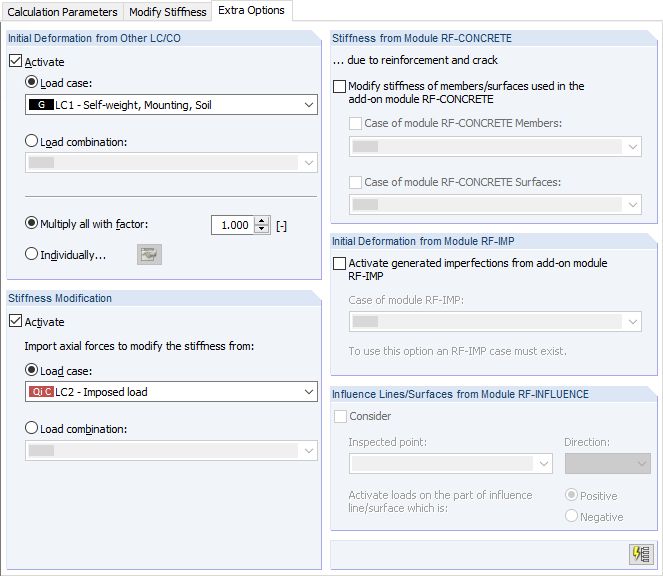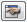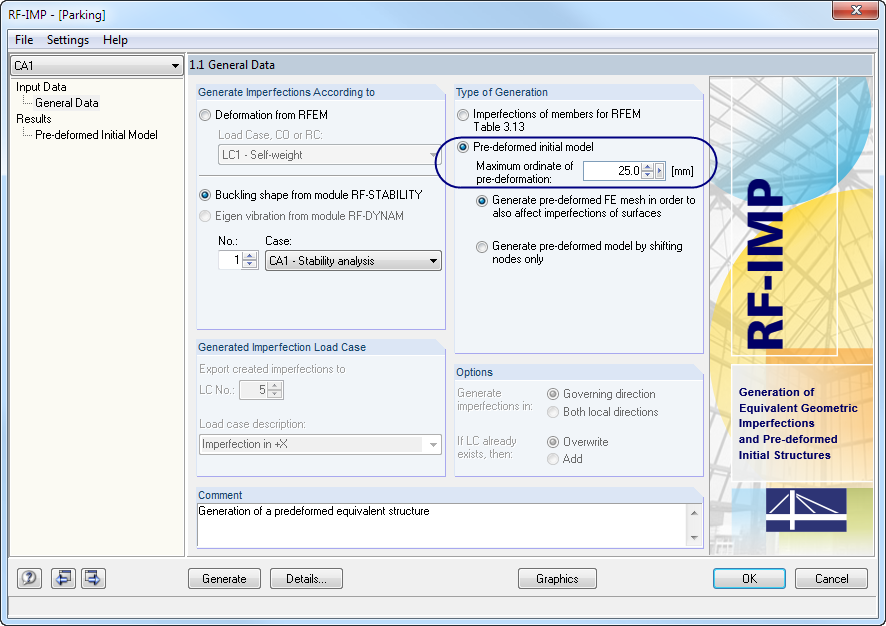# RFEM – Online Manual

Online manuals, introductory examples, tutorials, and other documentation.

# 7.3.1.3 Extra Options

#### Extra Options

This dialog tab is only displayed if the Extra options check box is selected in the Calculation Parameters dialog tab (see Figure 7.19).Figure 7.21 Extra Options dialog tab
Initial Deformation from Other LC/CO

Select a load case or load combination whose deformations you want to consider as the initial strain in the calculation. FE nodes are moved accordingly before the calculation starts. If no results are available for the selected load case or combination yet, they are automatically included in the calculation.

Specify the factor with which you want to scale the deformations:

• Multiply all with factor: Deformations of members, surfaces, and solids are globally multiplied by the specified factor.
• Individually: Thebutton opens a dialog box where a specific scaling factor of the deformation can be assigned to every member, every surface, and every solid.
Initial Forces for Geometric Stiffness

You can select a load case or load combination whose axial forces you want to use for an initial deformation. In this way, you can consider the stabilizing effect of a different load case (than the one specified above in the Initial Deformation dialog section), for example.

Access to this dialog section is only available for a calculation according to the second-order analysis.

Stiffness from Module RF-CONCRETE

Stiffnesses of reinforced concrete elements, which result from reinforcements and crack analyses according to the nonlinear design method performed in the RF-CONCRETE modules, can be taken into account in the calculation. After selecting the check box, specify the analysis case of the RF-CONCRETE Members or RF-CONCRETE Surfaces add-on module.

A calculation with the stiffnesses from RF-CONCRETE only succeeds if design cases have been created and if designs are possible without non-designable situations.

Initial Deformation from Module RF-IMP

Imperfections can be considered in the form of a pre-deformed equivalent model created in the RF-IMP add-on module. If the program is not licensed, equivalent imperfections for members (see Chapter 6.14) or initial deformations of a load case (see above) can be applied manually.

A calculation on the pre-deformed equivalent model is possible if the model was previously created in the RF-IMP add-on module: The add-on module generates imperfections on the basis of eigenvalues from RF-STABILITY, RF-DYNAM, or of deformations from an RFEM load case that are scaled to a maximum ordinate.Figure 7.22 RF-IMP add-on module with the Pre-deformed initial model type of generation

The equivalent model is stored separately without changing the actual geometrical data. The coordinates of FE nodes are only aligned with the equivalent model once load combinations are calculated.

You can use a different equivalent model for each load combination. Select the relevant case in the Case of module RF-IMP list.